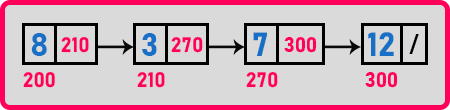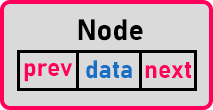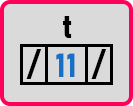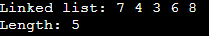# Doubly Linked List in C

## Doubly Linked List in C Language

In this article, we will give you an introduction to the Doubly Linked List in C Language. Please read our previous article, where we discussed Deleting a Node from a Circular Linked List in C Language with Examples. At the end of this article, you will understand Doubly Linked List with algorithms and examples using C Language.

##### Doubly Linked List in C Language

The linked list that we have seen in our previous articles can be called a singly linked list.In a singly linked list, every node will have a pointer to the next node.In a doubly linked list, a node is having a pointer to the next node as well as to the previous node. So it means from one node, we can go forward as well as we can come backward. We can access this list of elements bidirectional. We can traverse a doubly linked list in either direction.

Suppose we are starting from the first node i.e. 7. Then we can go to 4, then 3 and from 3 we can traverse in the backward direction i.e. 4, then 7. Like, inside a browser, if we open a website and we’ve clicked some links and then we go deeper then we can go back and forth afterward. Every new page you visit will be added to the doubly linked list structure so that you can move in between the pages.

If you take the example of the contact list on our mobile phones, there you can scroll up and down. You can move in both directions. So this is possible by using a doubly linked list. Now let us see how to define a node of the doubly linked list.

##### Doubly Linked List Node Structure:Node is having 3 elements or 3 data members i.e. data, prev (pointer to the previous node), and next (pointer to next node). Now we are defining the structure for this node by using C language.

struct Node{
int data;
struct Node *next;
struct Node *prev;
};

Data will hold your integer type number, next will point to the next node and prev will point to the previous node. Let us create a node and fill in some data in that node. For creating a node, we should have a pointer,

Struct Node *t;

‘t’ is a pointer. Now we assign ‘t’ to a new node.

t = (struct Node *) malloc (sizeof (struct Node));

This will assign a new node to the ‘t’ pointer. let us assign the data and pointers.

t->prev = NULL;
t->data = 11;
t->next = NULL;So a node will be created where t is pointing. You can create multiple nodes and create a linked list from those nodes. What are the operations that we can perform on a doubly linked list? We can perform the same operations as that we have done on a singly linked list i.e. display the linked list, count the number of nodes in the linked list, find max or min, etc. All the operations that we have performed are in the singly linked list, we can perform them here. Here just the structure is different. We can traverse in both directions.

The benefit of using a doubly linked list is we can access the data in either direction. Now let us look at the complete C program for the doubly linked list.

##### Example to understand Doubly Linked List in C Language:
```#include <stdio.h>
#include<stdlib.h>

struct Node {
struct Node * prev;
int data;
struct Node * next;
}* first = NULL;

void create(int A[], int n) {
struct Node * t, * last;
int i;

first = (struct Node * ) malloc(sizeof(struct Node));
first -> data = A;
first -> prev = first -> next = NULL;
last = first;

for (i = 1; i < n; i++) {
t = (struct Node * ) malloc(sizeof(struct Node));
t -> data = A[i];
t -> next = last -> next;
t -> prev = last;
last -> next = t;
last = t;
}
}

void Display(struct Node * p) {
while (p) {
printf("%d ", p -> data);
p = p -> next;
}
printf("\n");
}

int Length(struct Node * p) {
int len = 0;

while (p) {
len++;
p = p -> next;
}
return len;
}

int main() {
int A[] = {7, 4, 3, 6, 8};
create(A, 5);